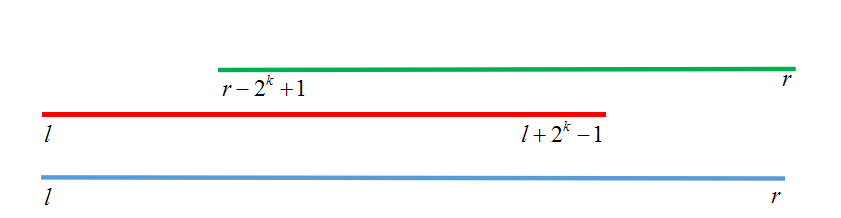浅谈ST表

ST表的功能很简单

算法

ST表是利用的是倍增的思想代码

#include<cstdio>
#include<cmath>
#include<algorithm>
using namespace std;
const int MAXN=1e6+10;
{
char c=getchar();int x=0,f=1;
while(c<'0'||c>'9'){if(c=='-')f=-1;c=getchar();}
while(c>='0'&&c<='9'){x=x*10+c-'0';c=getchar();}
return x*f;
}
int Max[MAXN];
int Query(int l,int r)
{
int k=log2(r-l+1);
return max(Max[l][k],Max[r-(1<<k)+1][k]);//把拆出来的区间分别取最值
}
int main()
{
#ifdef WIN32
freopen("a.in","r",stdin);
#endif
for(int j=1;j<=21;j++)
for(int i=1;i+(1<<j)-1<=N;i++)//注意这里要控制边界
Max[i][j]=max(Max[i][j-1],Max[i+(1<<(j-1))][j-1]);//如果看不懂边界的话建议好好看看图
for(int i=1;i<=M;i++)
{